# Dehn invariant

(diff) ← Older revision | Latest revision (diff) | Newer revision → (diff)
Quite generally, a scissors-congruence invariant assigns to a polytopein space an element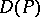in a group such that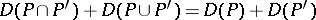,ifis degenerate, and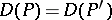if there is a motionof the space such that.
For the Dehn invariant, the group chosen is the tensor product. To a polytopewith edgesone associates the element, where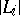is the length of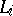and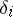is the dihedral angle of the planes meeting at. The Sydler theorem states that two polytopes in three-dimensional space are scissors equivalent if and only if they have equal volume and the same Dehn invariant, thus solving Hilbert's third problem in a very precise manner (cf. also Hilbert problems).